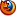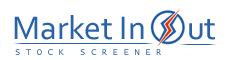Please enable JavaScript to view this page content properly Log In | Sign Up
 Pre-Tax Profit Margin Leverage Ratio Current Ratio Price / Book Ratio Price / Tangible Book Ratio Price / Cash Flow Ratio Price / Free Cash Flow Ratio Debt / Equity Ratio Price / Sales Ratio Income per Employee
 Price / Cash Flow Ratio Action Price / Cash Flow Ratio: More than 200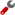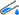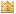Price / Cash Flow Ratio: 100 to 200Price / Cash Flow Ratio: 80 to 100Price / Cash Flow Ratio: 50 to 80Price / Cash Flow Ratio: 20 to 50Price / Cash Flow Ratio: 10 to 20Price / Cash Flow Ratio: 5 to 10Price / Cash Flow Ratio: 0 to 5Best viewed in